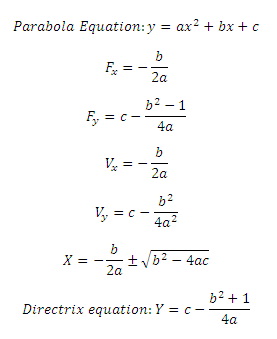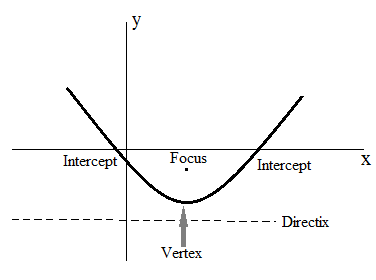# Parabola Calculator

This CalcTown calculator calculates calculates the focii, vertex, diretrix and intercepts of a parabola.

#### Result

m
m
m
m
mWhere,

a = co-efficent of X2

b = co-efficent of X

c =  constant term

Fx, Fy = Focus of the parabola

Vx ,Vy = Vertex of the parabola

X = Intercept length on x-axis